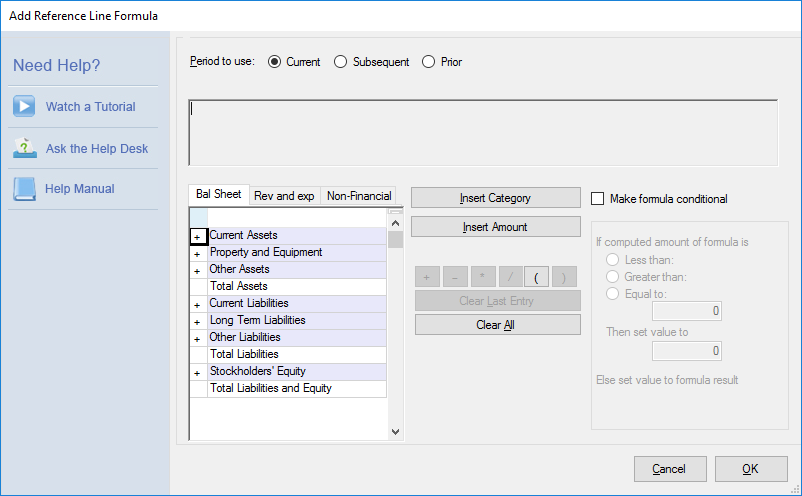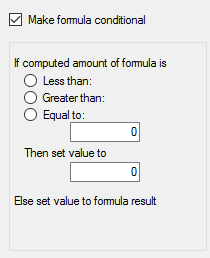# Formula Editor

We have overhauled the look of the formula builder in PlanGuru V8, in addition to adding some new functionality to it. To accommodate for this added functionality we have turned the formula builder into the formula wizard. Once you choose the formula option you will be taken to this screen:This still functions the same way as the old formula builder. You select your category using the window on the left hand side and click "Insert Category" to add it to the formula. We also now have the ability to add a conditional element to the formula by checking the "Make Formula Conditional" checkbox. Once you do that the conditional functionality will be unlocked.Using this you can dictate conditional terms in the formula. You can choose to have the formula set to a certain value depending on if it's result is less than, greater than, or equal to the results of the formula.